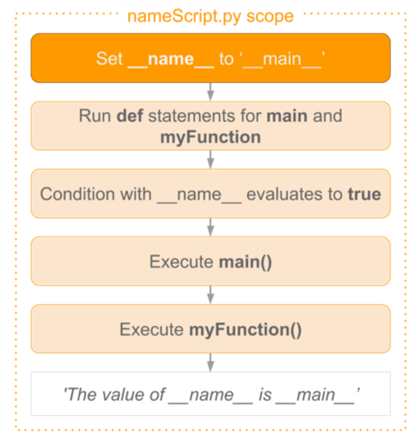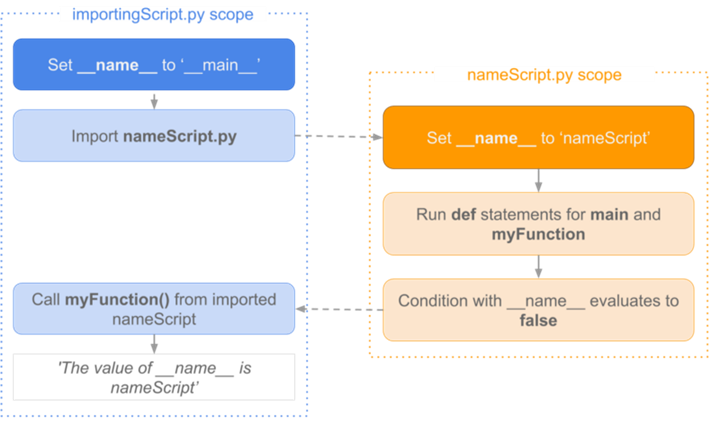In the process of learning Python, or reading other code written to it, you will encounter code like this.

 ``````1 2 `````` ``````if __name__ == "__main__": print("Hello World!") ``````We found that even if we remove the `if __name__ == '__main__'`, the program still runs as usual. Many of you only know that this is how it works, and you don’t look deeper into what exactly it does.

## Program entrance

For many programming languages, a program must have an entry point, such as C, C++, and the fully object-oriented programming languages Java, C#, and so on. If you have worked with these languages, the concept of a program entry point should be well understood. Similarly, Java and C# must have a main class that contains the Main method as the program entry point.

Python is different in that it is a scripting language, and instead of compiling the program into a binary before running it, it is dynamically interpreted line by line. That is, it runs from the first line of the script and has no uniform entry point.

if `__name__ == "__main__"`: is essentially an if judgment, but it is not a simple if judgment. That is, when the .py file is run, `__name__ `is `'__main__'` when the code under if is run, then no not run. Of course you can also write the code like this.

 ``````1 2 3 4 `````` ``````if __name__ == "__main__": print("Hello World!") else: print("Hello Mars!") ``````

## What is `__name__`?

name is a built-in Python variable that is a required property of every Python module, but its value depends on how you are executing this code.

• When you execute a script directly, the `__name__` variable of that script is equal to `'__main__'`
• When this script is imported into another program, the `__name__` variable is equal to the name of the script itself

### Scenario 1: Running the script directly

Suppose we have a `nameScript.py` with the following code.

 `````` 1 2 3 4 5 6 7 8 9 10 `````` ``````def myFunction(): print('The value of __name__ is ' + __name__) def main(): myFunction() if __name__ == '__main__': main() ``````

The flow after direct execution of this file is.The `__name__` variable is set to `'__main__'` before all other code is executed. After that, the bodies of the functions main() and myFunction() are loaded by executing the def statement. Then, because the expression after this if statement is true, the function main() is called. The main() function in turn calls myFunction(), which prints out the value of the variable `'__main__'`.

### Scenario 2: Import from other scripts

If you need to reuse this myFunction() function in another script, for example in importingScript.py, we can import `nameScript.py` as a module.

The content of `importingScript.py` is as follows.

 ``````1 2 3 `````` ``````import nameScript as ns ns.myFunction() ``````

At this point, we have two different scopes: one for importingScript and one for nameScript.In `importingScript.py`, the `__name__` variable is set to `'__main__'`. When importing nameScript, Python looks for a .py file with the corresponding name in the local path and in the path pointed to by the environment variable PATH, and when it finds it, it runs the code in the imported file.

This time, however, its own `__name__` variable is set to ’nameScript’ at import time, and as usual, the bodies of the functions main() and myFunction() are loaded. However, this time the expression after the if statement results in a false false, so the main() function is not called.

After the import is complete, go back to `importingScript.py`. Now that the function definition in the nameScript module has been imported into the current scope, we call the function in the module by means of ns.myFunction(), which returns the value of the variable ’nameScript’ inside the module.

If you try to print the value of the `__name__` variable in importingScript, then when you execute importingScript directly, it will also output `'__main__'`. The reason for this is that the variable is in the scope of importingScript.

### `__name__` can display the package path

We create a directory structure like this.

 ``````1 2 3 4 5 6 `````` ``````a ├── b │ ├── c.py │ └── __init__.py └── __init__.py d.py ``````

Code in the `c.py` file.

 ``````1 `````` ``````print(__name__) ``````

Code in the `d.py` file.

 ``````1 `````` ``````from a.b import c ``````

Running the `d.py` file results in the following.

 ``````1 `````` ``````a.b.c ``````

At this point, the `__name__` attribute of the `a.py` file becomes a.b.c, which exactly reflects the package path it is in.

## Code purpose: test the function in the module

Since a script’s own code is executed when it is introduced, we write a paragraph in each script if `__name__ == '__main__'`: If you want some code to be executed only when the script is executed directly, then put that code into an if statement block, the most common scenario is the test code.

 ``````1 2 3 4 5 6 7 8 9 `````` ``````def safe_division(a, b): if b == 0: return None return a/b if __name__ == '__main__': print(safe_division(10, 5) == 2) print(safe_division(10, 0) == None) ``````

After we write a function, we inevitably have to write some test code, and these test code we do not want them to be executed when we introduce them, but only when we actively execute for testing.